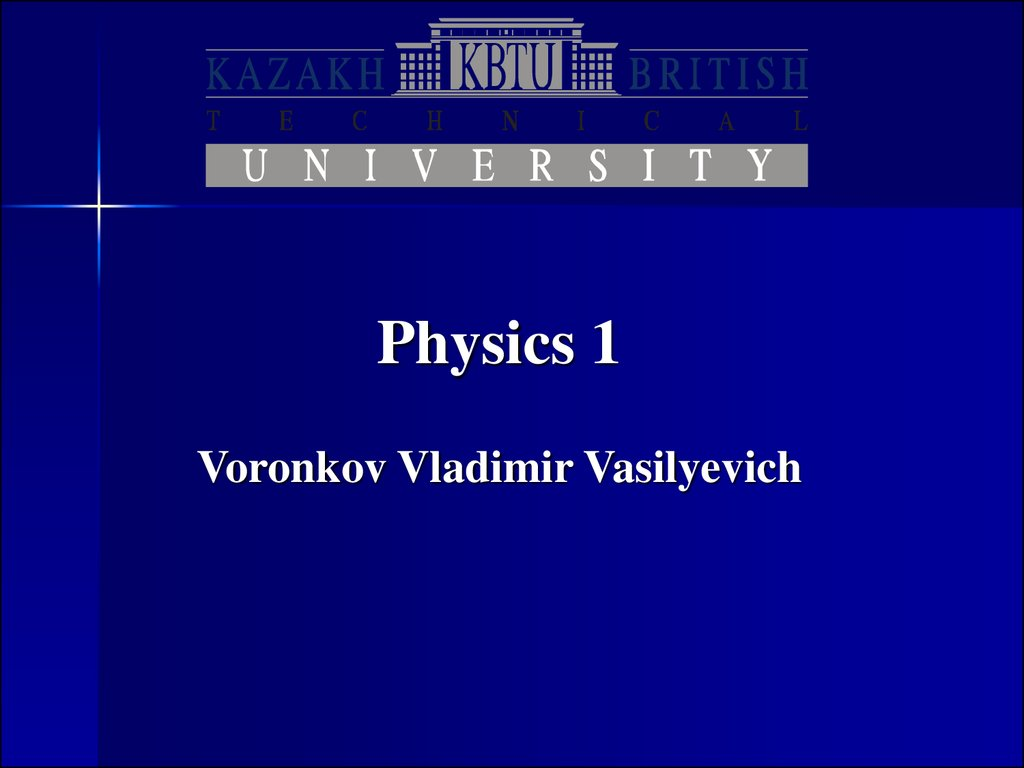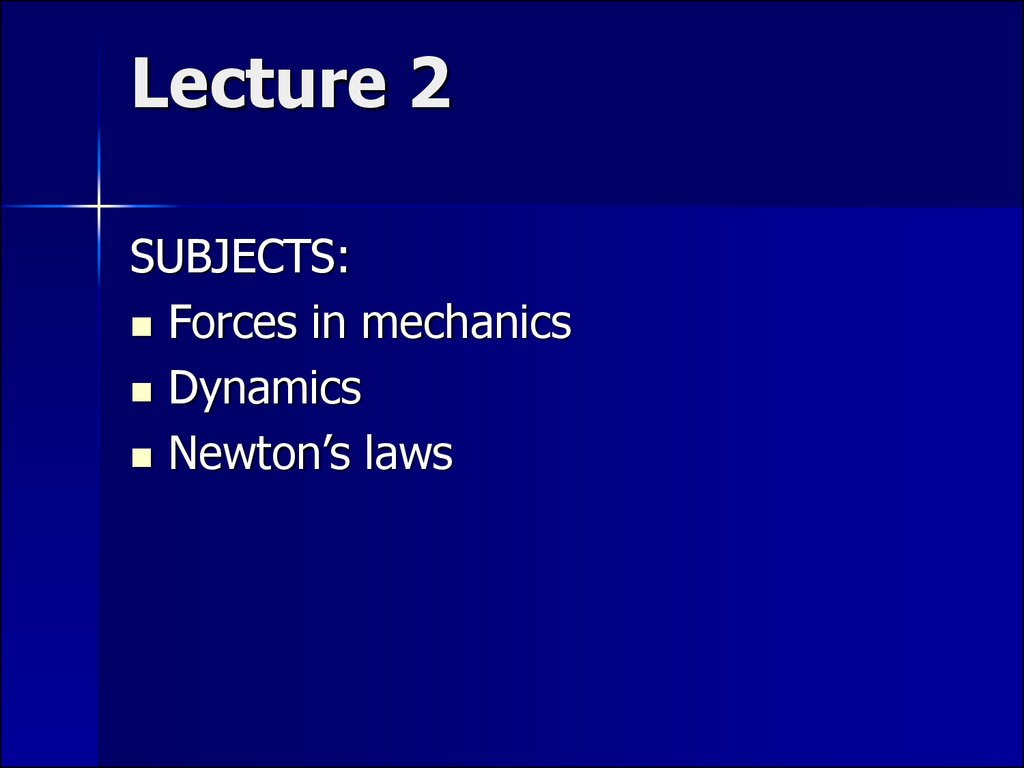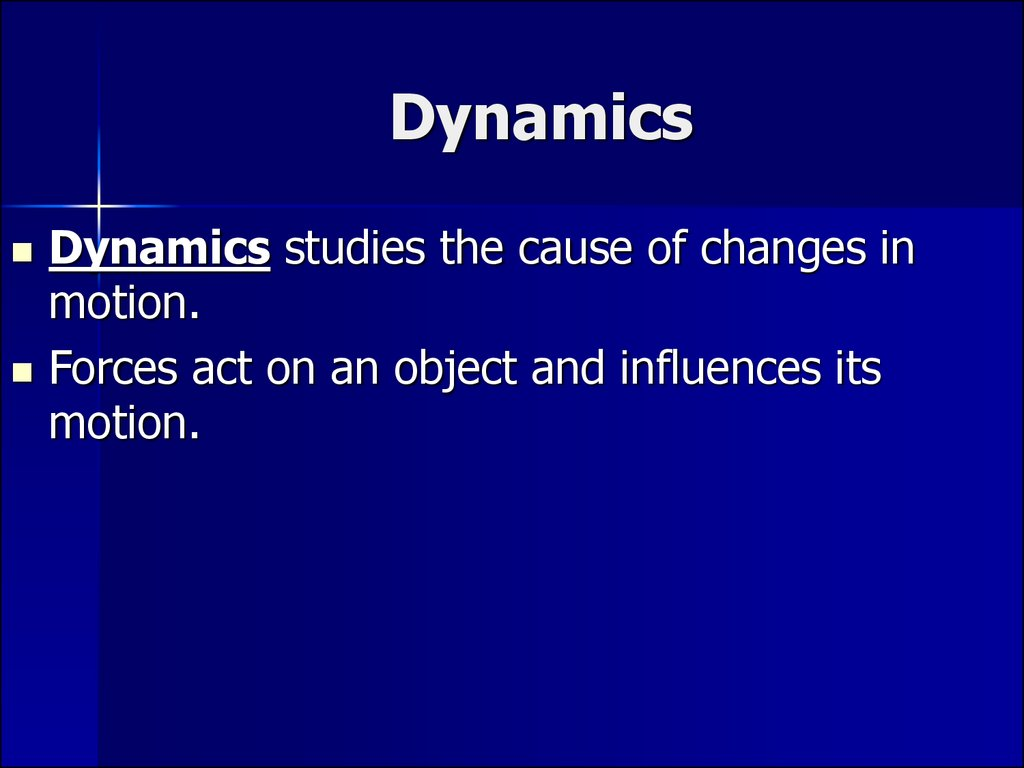# Subjects: forces in mechanics. Dynamics. Newton’s laws

Physics 1

## 2. Lecture 2

SUBJECTS:
Forces in mechanics
Dynamics
Newton’s laws

## 3. Dynamics

Dynamics studies the cause of changes in
motion.
Forces act on an object and influences its
motion.

## 4. Force

A force is a vector, so that it has:
– a direction
– a magnitude
Forces are additive, which means that
when several forces act together, the
subsequent motion of the object acted on
is the same as if a single force equal to the
vector sum of the individual forces were
acting. That single force is the net force:
Fnet Fi
i

## 7. Three fundamental forces

Gravitational force.
Electroweak force: a united force of
electromagnetism and the weak force,
responsible mainly for some types of radioactive
processes in nuclei and.
Strong force – a nuclear force.
The electromagnetic force is responsible for most
of the secondary forces: tension, friction, pull
and push forces, spring forces.

## 8. Newton’s first law of motion two equivalent variants:

1. When there is no net force acting on
an object, that object maintains its
motion with a constant velocity.
2. If an object does not interact with
other objects, it is possible to identify
a reference frame in which the object
has zero acceleration.

## 9.

Such a reference frame is called an
inertial frame of reference.
Newton’s first law does not say what
happens to an object with zero net
force; it says what happens in the
absence of a force. This is a subtle
but important difference that allows us
to define force as that which causes a
change in the motion. The
description of an object under the
effect of forces is covered by Newton’s
second law.

## 10. Newton’s second law of motion

When viewed from an inertial
reference frame, the acceleration of an
object is directly proportional to the
net force acting on it and inversely
proportional to its mass.

## 12. Newton’s third law of motion

When a force due to object B acts on
object A, then an equal and opposite
force due to object A acts on object B.

## 13. Mass and Weight

Mass is that property of an object that
specifies how much resistance an
object exhibits to changes in its
velocity. The greater the mass of an
object, the less that object accelerates
under the action of a given applied
force.

## 14.

The attractive force exerted by the Earth on an object is
called the gravitational force Fg. This force is directed
toward the center of the Earth, and its magnitude is
called the weight of the object.
Fg mg
Thus, the weight of an object, being defined as the
magnitude of Fg , is equal to mg. Because it depends
on g, weight varies with geographic location. Because it
decreases with increasing distance from the center of
the Earth, objects weigh less at higher altitudes than at
sea level.

## 15. Mass and weight are two different quantities

The weight of an object is equal to
the magnitude of the gravitational
force exerted on the object and
varies with location. For example, a
person who weighs 180 lb on the
Earth weighs only about 30 lb on
the Moon. On the other hand, the
mass of an object is the same ever
everywhere: an object having a
mass of 2 kg on the Earth also has a
mass of 2 kg on the Moon.

## 16. Action – Reaction forces

Forces always occur in pairs, or that a
single isolated force cannot exist. The
force that object 1 exerts on object 2
may be called the action force and the
force of object 2 on object 1 the
reaction force. In reality, either force
can be labeled the action or reaction
force. The action force is equal in
magnitude to the reaction force and
opposite in direction. In all cases, the
action and reaction forces act on
different objects and must be of the
same type.

Force
F
Acceleration a,g
N=kg*m/s2
m/s2